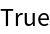Function Repository Resource:

Perform a folding operation parsing multiple arguments

Contributed by: Sander Huisman
 ResourceFunction["FoldThreadList"][f,x ,{{a1,a2,…},{b1,b2,…},…}] gives {x, f[x,a1,b1,…], f[f[x,a1,b2,…], a2,b1, …], …}. ResourceFunction["FoldThreadList"][f,{{a1,a2,…},{b1,b2,…},…}] gives {{a1,b1,…},f[{a1,b1,…},a2,b2,…],f[f[{a1,b1,…},a2,b2,…],a3,b3,…],…}. ResourceFunction["FoldThreadList"][f] represents an operator form of ResourceFunction["FoldThreadList"] that can be applied to expressions.

Examples

Basic Examples

Fold a function over lists, threading the elements:

 In:=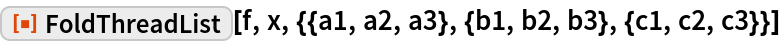Out=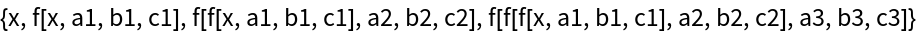Scope

Without an initializer, the first elements of list is the initializer:

 In:=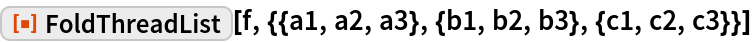Out=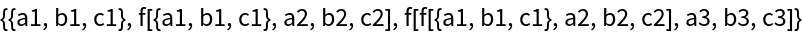Make an operator:

 In:=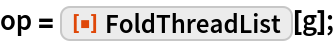Feed a list of lists:

 In:=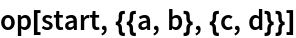Out=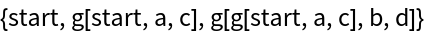Applications

First integrate x from 0 to 2, then y from 0 to 2, then z from 0 to 2:

 In:=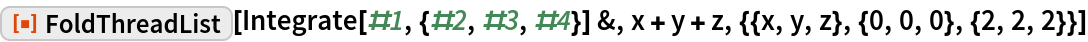Out=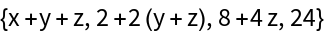Create a continued fraction with different numerators:

 In:=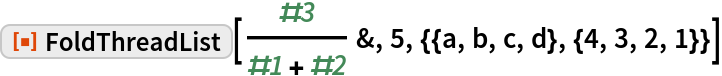Out=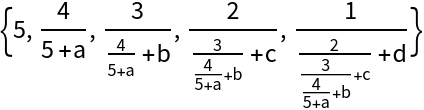Properties and Relations

FoldList and FoldThreadList are related for the case in which a single argument is folded in:

 In:=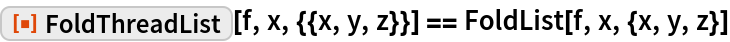Out=